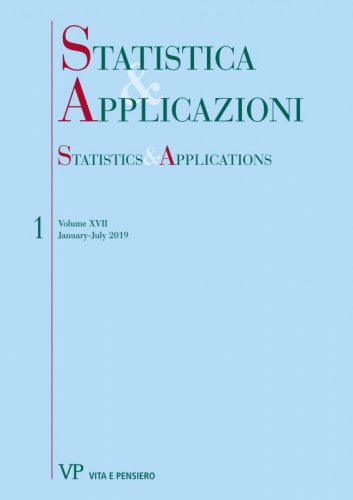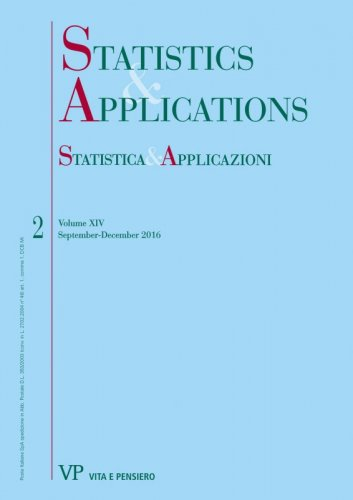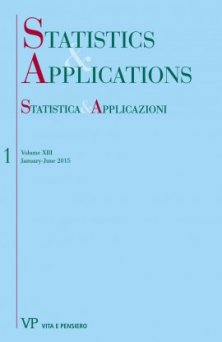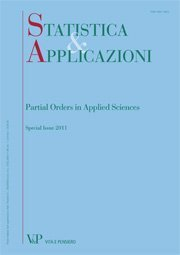Libri di Lucio De Capitani - libri Statistica & Applicazioni

# Lucio De Capitani

### Titoli dell'autore

Asymptotic Confidence Intervals for Parameters Estimated through the Ratio of Asymptotically Normal Statistics digitalYear: 2019
In this paper, four different approaches for the definition of asymptotic confidence intervals for the ratio of two unknown parameters are reviewed and compared via a simulation study. The considered approaches are based on the well known Delta Method and on the distribution of the ratio of correlated normal random variables...
Some remarks on Zenga’s approach to kurtosis digitalYear: 2016
In this paper, several insights on the Zenga’s approach for the measurement of Kurtosis are provided. These insights mainly regard the connections between Kurtosis and Concentration indexes and the relation between the Kurtosis diagram and an extension of the well-known Lorenz curve, i.e. the relative first incomplete moment function...
On the distribution of the sum of cograduated discrete random variables with applications to credit risk analysis digitalYear: 2015
This paper focuses on the notion of cograduation which was first introduced in 1939 by the Italian statistician Tommaso Salvemini. In few words, a certain number of random variables are cograduated if they are associated with the maximum positive dependence. Here, it is shown how to derive the probability distribution of the sum of cograduated discrete random variables...
The confluent hypergeometric-mixture of Polisicchio distributions: a generalized Zenga distribution
Free
digitalYear: 2013
We propose a generalization of the three-parameters Zenga distribution obtaining a four-parameters model. The generalization is performed using the confluent hypergeometric distribution as mixing distributions in place of the classical beta. We compare the flexibility of the resulting model with that of the Zenga distribution observing some improvements.
Point and interval estimation for some financial performance measures
Free
digitalYear: 2011
We study the estimators of three financial performance measures: the Sharpe Ratio, the Mean Difference Ratio and the Mean Absolute Deviation Ratio. The analysis is performed under two sets of assumptions. First, the case of i.i.d. Normal returns is considered. After that, relaxing the normality assumption, the case of i.i.d. returns is investigated. In both situations, we study the bias of the estimators and we propose their bias-corrected version. The exact and asymptotic distribution of the three estimators is derived under the assumption of i.i.d. Normal returns. Concerning the case of i.i.d. returns, the asymptotic distribution of the estimators is provided. The latter distributions are used to define exact or asymptotic confidence intervals for the three indices. Finally, we perform a simulation study in order to assess the efficiency of the bias corrected estimators, the coverage accuracy and the length of the asymptotic confidence intervals. Keywords: Financial Performance Measure, Sharpe Ratio, Mean Difference Ratio, Mean Absolute Deviation Ratio, Concentration Measures, Statistical Analysis of Financial Data.